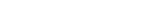MathExamle.com# Addition Subtraction Multiplication Division of fractions

Create worksheets of mathematical examples with addition, subtraction, multiplication and division of fractions, select type of a generator and viewing some samples of task

Creates examples with operations of addition, subtraction, multiplication and division of fractions, the answers are always positive

2 Variants 3 Levels
 3 × 1 10 + 37 = 5 51 255
 74 569 – 3 × 2701 = 724 4 543
Variant: 1 Level: Hard

Creates examples with operations of addition, subtraction, multiplication and division of fractions, the answers can be negative

2 Variants 3 Levels
 – 11 × 57 – 7 = 19 143 26
 1 ÷ 5 + 14 491 = 2 704 635
Variant: 1 Level: Hard